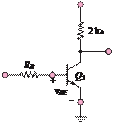### Create an Account

Home / Questions / 1044 Show that the circuit of Figure P1043 functions as a NAND gate if the output is...

# 1044 Show that the circuit of Figure P1043 functions as a NAND gate if the output is taken at vo2 1045 In Figure P1045 the minimum value of vin for a high input is 20 V Assume that

10.44      Show that the circuit of Figure P10.43 functions as a NAND gate if the output is taken at vo2.

10.45      In Figure P10.45, the minimum value of vin for a high input is 2.0 V. Assume that transistor Q1 has a β of at least 10. Find the range for resistor Rthat can guarantee that the transistor Q1 is on.+VCC = 5 V

vout

vin

Jun 18 2020 View more View LessSubscribe To Get Solution